# Light - Reflection and Refraction - NCERT Questions

Q 1.

Define the principal focus of a concave mirror.

SOLUTION:

A point on the principal axis where the parallel rays of light after reflecting from a concave mirror meet.

Q 2.

The radius of curvature of a spherical mirror is 20 cm. What is its focal length ?

SOLUTION:

Radius of curvature, R = 20 cm
∴ Focal length,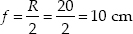Q 3.

Name a mirror that can give an erect and enlarged image of an object.

SOLUTION:

A concave mirror gives an erect and enlarged image of an object held between pole and principal focus of the mirror.

Q 4.

Why do we prefer a convex mirror as a rear-view mirror in vehicles?

SOLUTION:

This is because a convex mirror forms an erect and diminished (small in size) images of the objects behind the vehicle and hence the field of view behind the vehicle is increased.

Q 5.

Find the focal length of a convex mirror whose radius of curvature is 32 cm.

SOLUTION:

Given, R = + 32 cm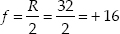Thus, the focal length of the convex mirror = + 16 cm.

Q 6.

A concave mirror produces three times magnified real image of an object placed at 10 cm in front of it. Where is the image located ?

SOLUTION:

Here, linear magnification (m) = – 3
(Negative sign for real image, which is inverted)
Object distance (u) = – 10 cm
Image distance (v) = ?
As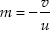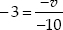v = –30 cm
The image is located at 30 cm in front of the mirror.

Q 7.

The image formed by a concave mirror is observed to be virtual, erect and larger than the object. Where should be the position of the object? (A) Between the focus and the centre of curvature (B) At the centre of curvature (C) Beyond the centre of curvature (D) Between the pole of the mirror and its focus.

SOLUTION:

For virtual, erect and larger image, the object must lie between the pole of the mirror and its focus.

Q 8.

No matter how far you stand from a mirror, your image appears erect. The mirror is likely to be (A) plane (B) concave (C) convex (D) either plane or convex

SOLUTION:

The image is erect in a plane mirror and also in a convex mirror, for all positions of the object.

Q 9.

We wish to obtain an erect image of an object, using a concave mirror of focal length 15 cm. What should be the range of distance of the object from the mirror? What is the nature of the image? Is the image larger or smaller than the object? Draw a ray diagram to show the image formation in this case.

SOLUTION:

A concave mirror produces an erect image if the object is placed between the pole and the focus of the concave mirror. Thus, object may be placed at any position whose distance is less than 15 cm from the concave mirror. The image is virtual and erect. The image is larger than the object. The ray diagram is shown below.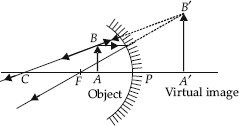Q 10.

Name the type of mirror used in the following situations. (A) Headlights of a car (B) Side/rear-view mirror of a vehicle (C) Solar furnace

SOLUTION:

(A) For head lights of a car, we use a concave mirror. The light source is held at the focus of the mirror. On reflection, a strong parallel beam of light emerges.
(B) A convex mirror is used as side rear view mirror, because its field of view is larger and it forms virtual, erect and diminished images of objects behind.
(C) For solar furnace, we use a concave mirror. Light from the sun, on reflection from the mirror, is concentrated at the focus of the mirror, producing heat.

Q 11.

An object is placed at a distance of 10 cm from a convex mirror of focal length 15 cm. Find the position and nature of the image.

SOLUTION:

Here, object distance (u) = –10 cm,
focal length (f) = 15 cm,
image distance (v) = ?
As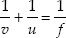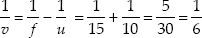v = 6 cm
Here, + sign of v indicates that image is at the back of the mirror. It must be virtual, erect and smaller in size than the object

Q 12.

The magnification produced by a plane mirror is m = + 1. What does this mean?

SOLUTION:

As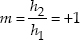h2 = h1
i.e., size of image is equal to size of the object. Further, + sign of m indicates that the image is erect and hence virtual.

Q 13.

An object 5.0 cm in length is placed at a distance of 20 cm in front of a convex mirror of radius of curvature 30 cm. Find the position of the image, its nature and size.

SOLUTION:

Here, object size (h1) = 5.0 cm, object distance (u) = –20 cm
Radius of curvature (R) = 30 cm, image distance (v) = ?
As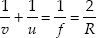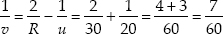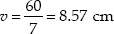Positive sign of v indicates that image is at the back of the mirror. It must be virtual and erect.
As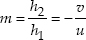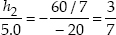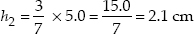This is the size of the erect image.

Q 14.

An object of size 7.0 cm is placed at 27 cm in front of a concave mirror of focal length 18 cm. At what distance from the mirror should a screen be placed, so that a sharp focussed image can be obtained? Find the size and the nature of the image.

SOLUTION:

Here, object size (h1) = 7.0 cm, object distance
(u) = – 27 cm
focal length (f) = –18 cm,
image distance (v) = ?
As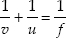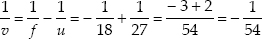v = – 54 cm
Therefore, the screen should be held in front of the mirror at a distance of 54 cm from the mirror. The image obtained on the screen will be real.
As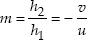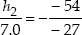h2 = – 14.0 cm
Negative sign of h2 shows that the image is inverted.

Q 15.

A ray of light travelling in air enters obliquely into water. Does the light ray bend towards the normal or away from the normal? Why?

SOLUTION:

When a ray of light travels from air into water obliquely, it bends towards the normal. This is because water is optically denser than air. On entering water, speed of light decreases and the light bends towards normal.

Q 16.

Light enters from air to glass having refractive index 1.5. What is the speed of light in glass? The speed of light in vacuum is 3 × 108m s–1.

SOLUTION:

Here, refractive index, n = 1.5, speed of light in vacuum = c = 3 × 108 m s–1
speed of light in glass, v = ?
From,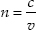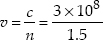= 2 × 108 m s–1

Q 17.

Give the medium having highest optical density. Also, give the medium with lowest optical density.

SOLUTION:

The medium with highest optical density is diamond and its refractive index is maximum (= 2.42), also, the medium with lowest optical density is air and its refractive index is minimum (= 1.0003).

Q 18.

You are given kerosene, turpentine and water. In which of these does the light travel fastest?

SOLUTION:

We know from the definition of refractive index, that the speed of light is higher in a medium with lower refractive index. So, the light travels fastest in water relative to kerosene and turpentine.

Q 19.

The refractive index of diamond is 2.42. What is the meaning of this statement?

SOLUTION:

This statement means that the speed of light in diamond is lower by a factor of 2.42 relative to that in vacuum.

Q 20.

Define 1 dioptre of power of a lens.

SOLUTION:

The power of a lens whose focal length is one metre (1 m) is one dioptre.

Q 21.

A convex lens forms a real and inverted image of a needle at a distance of 50 cm from it. Where is the needle placed in front of the convex lens if the image is equal to the size of the object? Also, find the power of the lens.

SOLUTION:

Distance of the image from the lens, v = 50 cm
Distance of the object from the lens, u = ?
Size of the image, I = Size of the object, O
From the definition, if h is the height of the image and that of the object,
magnification =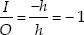[∵ The image is inverted]
For a lens, magnification =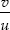So,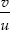= –1 ⇒ u = –v = – 50 cm
So, the needle (the object) is placed at a distance of 50 cm in front of the lens.
Using the lens formula,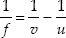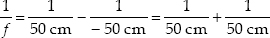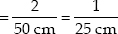f = 25 cm
Then, power of the lens =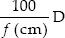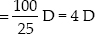Q 22.

Find the power of a concave lens of focal length 2 m.

SOLUTION:

Focal length of the concave lens = –2 m
So, power of the concave lens =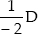= –0.5 D

Q 23.

Which one of the following materials cannot be used to make a lens ? (A) Water (B) Glass (C) Plastic (D) Clay

SOLUTION:

The correct answer is (D), because clay is opaque.

Q 24.

Which of the following lenses would you prefer to use while reading small letters found in a dictionary? (A) Convex lens of focal length 50 cm (B) A concave lens of focal length 50 cm (C) A convex lens of focal length 5 cm (D) A concave lens of focal length 5 cm

SOLUTION:

For reading small letters in a dictionary, we need to use a convex lens of smaller focal length. Choice (C) is correct.

Q 25.

One half of a convex lens is covered with a black paper. Will this lens produce a complete image of the object ? Verify your answer experimentally. Explain your observations.

SOLUTION:

Yes, it will produce a complete image of the object, as shown in figure. This can be verified experimentally by observing the image of a distance object like tree on a screen, when lower half of the lens is covered with a black paper. However, the intensity or brightness of image will reduce.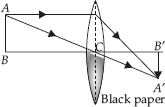Q 26.

An object 5 cm in length is held 25 cm away from a converging lens of focal length 10 cm. Draw the ray diagram and find the position, size and the nature of the image formed.

SOLUTION:

Here, object size, h1 = 5 cm
object distance, u = – 25 cm
focal length of lens, f = 10 cm
image distance, v = ?
image size, h2 = ?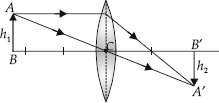As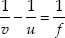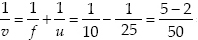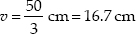As v is positive, the image formed is real; on the right side of the lens, as shown in figure above.
As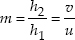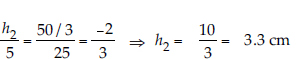Negative sign shows that the image is inverted.

Q 27.

A concave lens of focal length 15 cm forms an image 10 cm from the lens. How far is the object placed from the lens? Draw the ray diagram.

SOLUTION:

Here, focal length of lens, f = – 15 cm
image distance, v = – 10 cm
object distance,
u = ?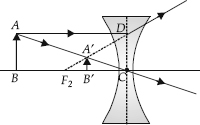As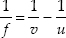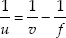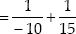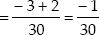u = – 30 cm

Q 28.

Find the focal length of a lens of power –2.0 D. What type of lens is this ?

SOLUTION:

Here, focal length, f = ?, power, P = –2.0 D
As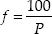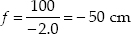As power of lens is negative, the lens must be concave.

Q 29.

A doctor has prescribed a corrective lens of power +1.5 D. Find the focal length of the lens. Is the prescribed lens diverging or converging?

SOLUTION:

Power of the lens, P = +1.5 D = + 1.5 m–1
∴ Focal length of the lens, f =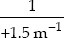= 0.667 m = 66.7 cm
Thus, the focal length of the lens is 66.7 cm
Since the focal length of the lens is positive, hence the given lens is a converging lens.